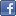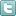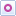EE Times-India > EDA/IP

EDA/IP# A primer on C-slow retiming, system hyper pipelining

Posted: 25 Apr 2014Print Version

Keywords:pipelining  C-slow retiming  CPU  RTL  verification

We can think of the two levels of AND as implementing a simple equation or algorithm. In the image above, this equation is solved (or evaluated) in a single clock cycle. An alternative approach would be to add some register elements and solve the equation in two clock cycles, as illustrated in figure 3.

The logical result is identical for both circuits. However, in this new circuit, we can start a completely independent calculation on the second clock cycle. Also, if we assume that each AND gate represents multiple levels of combinatorial logic, we can theoretically run the clock at twice the speed, so the time required to solve a single equation does not change. Looking at this another way, by adding registers, we can solve the same equation twice as often.Figure 3: Solving the same equation in two cycles.

A design example
Let's apply this technique to a slightly more sophisticated design. Any single-clock design can be defined as a set of inputs, a set of outputs, and a graph of logic elements and registers.

CSR can automatically perform appropriate register insertion on our more sophisticated design, as illustrated in figure 5.

In this case, it takes two clock cycles to achieve the same behaviour as the original design, but we now have a second, totally independent design that uses the combinatorial logic in a time-sliced fashion.Figure 4: Simplified representation of a single-clock design.

Whether the original design is already pipelined (as in a CPU) is totally irrelevant. If we follow the rule to insert the same number of registers in any of the original logic paths, we multiply the functionality of the design/core. If the registers are placed using a timing-driven algorithm, the performance of a single core remains almost the same. More register levels can be inserted as required, and the functionality multiplies accordingly. Performing this automatic register insertion on the RTL simplifies the entire implementation and verification process.Figure 5: Single-clock design after register insertion.

Timing estimation on RTL
No matter which Altera or Xilinx component families I use (e.g., Flex10k or Virtex), this is the central observation that facilitates my work on the CSR technology on RTL. Now, what do you think Johann Carl Friedrich Gauss might have to do with timing estimation on a Virtex 5 FPGA in 2014?

 Related Articles Editor's Choice
Comment on "A primer on C-slow retiming, system ..."

Top Ranked Articles

WebinarsVisit Asia Webinars to learn about the latest in technology and get practical design tips.

Search EE Times India
Services

Go to topConnect on FacebookFollow us on TwitterFollow us on Orkut
﻿

Back to Top